# Working with Meshes in Drawings

## Yurii Pylnyk

March 21, 2019

A mesh (OdDbSubDMesh) is a set of vertices that form a surface. A mesh includes features such as color and normals.

With color you can set the color of individual elements of a mesh (face, vertex).

• OdResult setVertexNormalArray(OdGeVector3dArray& arrNorm) method — Provides an array of vertex normals.
• OdResult getNormalArray (OdGeVector3dArray& normalArray) const method — Returns (calculates) a Level 0 (base) vertex normal array.
• OdResult getSubDividedNormalArray(OdGeVector3dArray& normalArray) const — Returns (calculates) a smoothed mesh vertex normal array.

For normals, the methods getNormalArray(…) and getSubDividedNormalArray(…) calculate the normal for all vertices. Also, you can provide an array of vertex normals, which are calculated manually (using setVertexNormalArray(…)). But the normals should map one to one with the control points of a level zero mesh. The length of the vertex normal array needs to match the length of the control point array.

The method getNormal of the class OdDbSubDMesh allows you to calculate normals on the mesh-surface. Here is example source code:

``````OdDbSubDMeshPtr pMesh = OdDbSubDMesh::createObject();
...
pMesh->setCylinder(90., 60., 90., 3, 3, 3, 4);
OdGeVector3dArray normals;
pMesh->getSubDividedNormalArray(normals);
pMesh->setVertexNormalArray(normals);
...
``````

The result of executing this source code is shown below. The mesh (shaded mode) on the left doesn’t have normals; the mesh on the right has normals and, as a result, we have a smooth surface.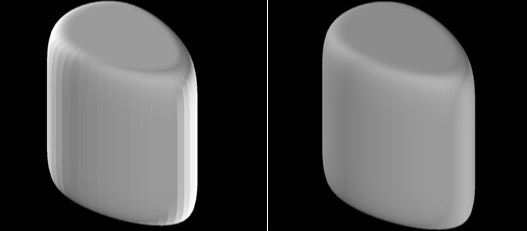The mesh (OdDbSubDMesh) has five levels of smoothness from 0 to 4. You can redefine (reassign) the level of smoothness. When the level of smoothing is applied to a surface and the subdRefine() method is invoked, all surface properties are recalculated (vertices, edges, faces). Also the surface receives a new level of smoothing (for example 4) and the level is accepted as zero.

Example source code:

``````…
for (int levSmooth = 0; levSmooth <= 4; levSmooth++)
{
OdDbSubDMeshPtr pMesh = OdDbSubDMesh::createObject();
pMesh->setCylinder(90., 60., 90., 3, 3, 3, levSmooth);
res = pMesh->subdRefine();
}
…
``````

When the created mesh is opened and rendered, the result is shown below with level smoothing from 0 to 4.

Wireframe mode: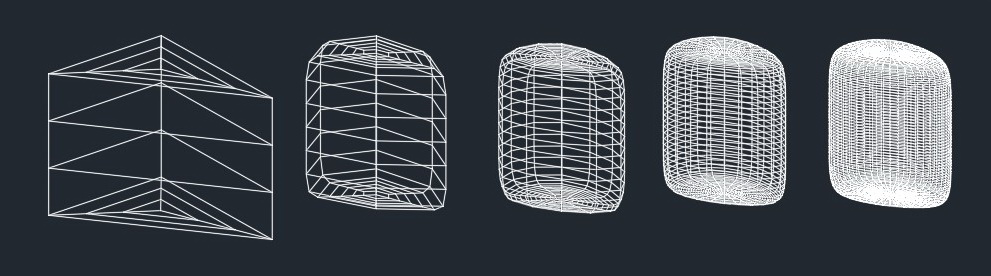Realistic mode: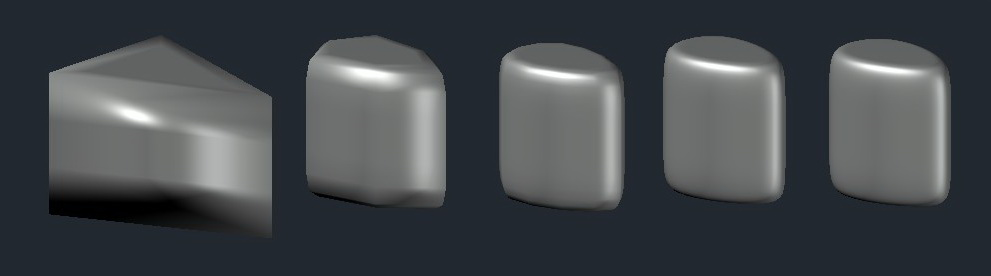The mesh can be converted to a surface. These methods allow you to convert a specified entity to a surface:

``````OdResult convertToSurface (bool bConvertAsSmooth, const OdDbSubentId& id, OdDbSurfacePtr& pSurface) const;
-	bool bConvertAsSmooth – isn`t supported yet.
-	const OdDbSubentId& id – specified entity, which will be converted to surface.
-	OdDbSurfacePtr& pSurface – resulting surface.

OdResult convertToSurface (bool bConvertAsSmooth, bool optimize, OdDbSurfacePtr& pSurface) const;
-	bool bConvertAsSmooth, bool optimize – isn`t supported yet.
-	OdDbSurfacePtr& pSurface – resulting surface.
``````

Also, a mesh can be converted to a 3D solid. The API method of OdDbSubDMesh is shown below:

``````OdResult convertToSolid (bool bConvertAsSmooth, bool optimize, OdDb3dSolidPtr& pSolid) const;
-	bool bConvertAsSmooth, bool optimize – isn`t supported yet.
-	OdDb3dSolidPtr& pSolid – resulting solid.
``````

The OdDbSubDMesh API allows you to create standard primitives (box, sphere, cylinder, pyramid, wedge, cone, torus). Here are code examples and results of drawing these operations:

``````…
OdDbSubDMeshPtr pMesh = OdDbSubDMesh::createObject();
pMesh->setBox(30, 30, 30, 3, 3, 3, 0);
OdDbSubDMeshPtr pMesh1 = OdDbSubDMesh::createObject();
pMesh1->setSphere(30., 10, 10, 4);
OdDbSubDMeshPtr pMesh2 = OdDbSubDMesh::createObject();
pMesh2->setCone(30., 30., 30., 3, 3, 3, .0, 4);
OdDbSubDMeshPtr pMesh3 = OdDbSubDMesh::createObject();
pMesh3->setPyramid(90., 60., 3, 3, 3, 3, .0, 0);
OdDbSubDMeshPtr pMesh4 = OdDbSubDMesh::createObject();
pMesh4->setTorus(90., 3, 3, .2, 0, 4);
OdDbSubDMeshPtr pMesh5 = OdDbSubDMesh::createObject();
pMesh5->setWedge(100., 100., 100., 2, 2, 2, 2, 2, 0);
…``````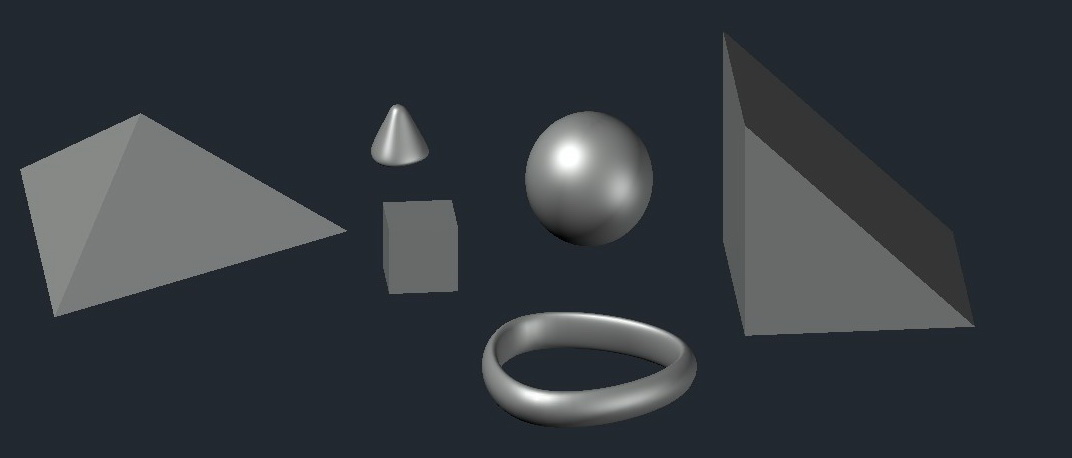Wireframe mode: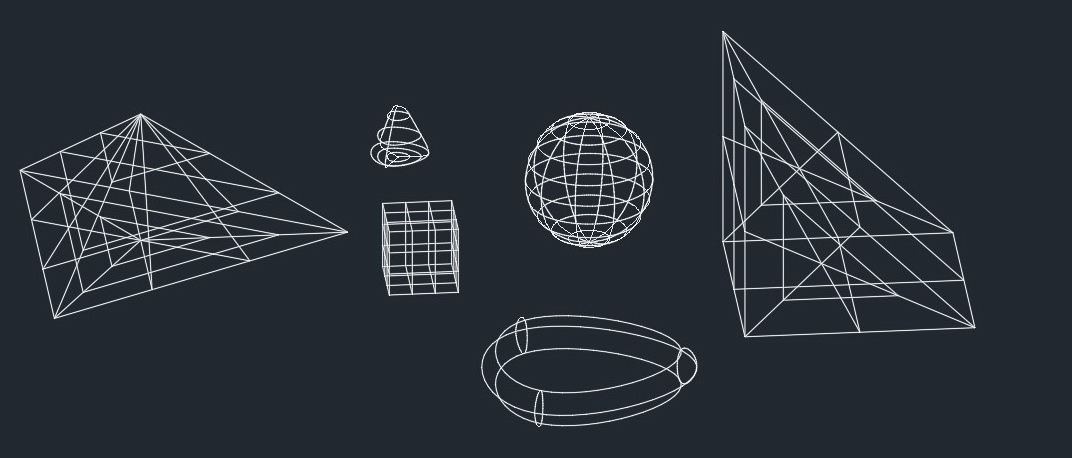When the smoothing level increases, the сount of vertices and faces increases too. Below is a code example and the result of rendering.

``````… database preparation
for (int levSmooth = 0; levSmooth <= 4; levSmooth++)
{
OdDbSubDMeshPtr pNew = OdDbSubDMesh::createObject();
OdGePoint3dArray vertexArray;
OdInt32Array faceArray;

vertexArray.append(OdGePoint3d(0, 0, 0));
vertexArray.append(OdGePoint3d(1, 0, 0));
vertexArray.append(OdGePoint3d(1, 1, 0));
vertexArray.append(OdGePoint3d(2, 2, 0));

faceArray.append(3);
faceArray.append(0);
faceArray.append(1);
faceArray.append(2);

pNew->setSubDMesh(vertexArray, faceArray, levSmooth); // eOk
pNew->subdRefine();
... saving database
}
``````

Note: When the method subdRefine() is called, the mesh is recalculated to corresponding smoothness, but the current level of smoothness is assigned as base (0).

Wireframe mode: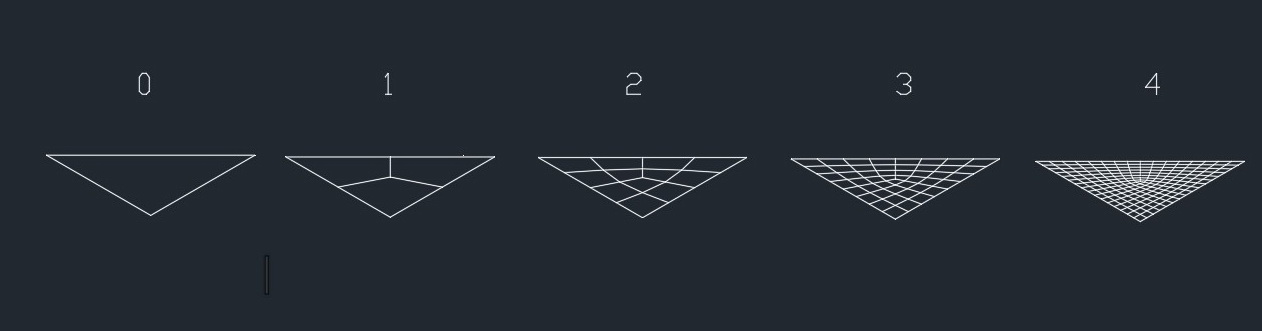You can see that the count of faces and vertices is changed because of the smoothness (invoked subdRefine()):
Level 0: vertices(3), faces(1);
Level 1: vertices(8), faces(3);
Level 2: vertices(20), faces(12);
Level 3: vertices(62), faces(48);
Level 4: vertices(218), faces(192);